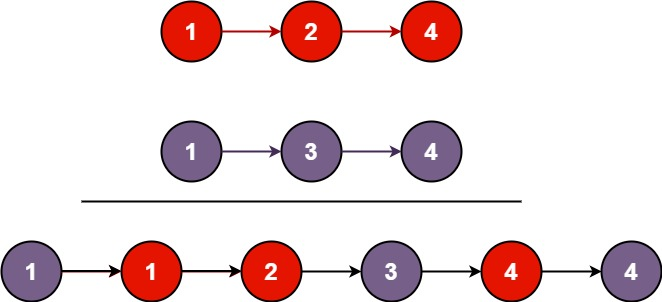## 21. Merge Two Sorted Lists

Merge two sorted linked lists and return it as a sorted list. The list should be made by splicing together the nodes of the first two lists.

Example 1:```Input: l1 = [1,2,4], l2 = [1,3,4]
Output: [1,1,2,3,4,4]
```

Example 2:

```Input: l1 = [], l2 = []
Output: []
```

Example 3:

```Input: l1 = [], l2 = 
Output: 
```

Constraints:

• The number of nodes in both lists is in the range `[0, 50]`.
• `-100 <= Node.val <= 100`
• Both `l1` and `l2` are sorted in non-decreasing order.

## Rust Solution

``````struct Solution;
use rustgym_util::*;

impl Solution {
fn merge_two_lists(l1: ListLink, l2: ListLink) -> ListLink {
if l1.is_none() {
return l2;
}
if l2.is_none() {
return l1;
}
let mut p1 = l1.unwrap();
let mut p2 = l2.unwrap();
if p1.val < p2.val {
p1.next = Self::merge_two_lists(p1.next, Some(p2));
Some(p1)
} else {
p2.next = Self::merge_two_lists(Some(p1), p2.next);
Some(p2)
}
}
}

#[test]
fn test() {
let a = list!(1, 2, 4);
let b = list!(1, 3, 4);
let c = list!(1, 1, 2, 3, 4, 4);
assert_eq!(Solution::merge_two_lists(a, b), c);
}
``````

Having problems with this solution? Click here to submit an issue on github.